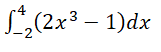# FTCE Mathematics 6-12 (026): Practice & Study Guide Final Exam

Free Practice Test Instructions:

Choose your answer to the question and click 'Continue' to see how you did. Then click 'Next Question' to answer the next question. When you have completed the free practice test, click 'View Results' to see your results. Good luck!

#### Question 2 2. What is the solution for the given equation below?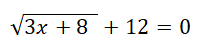#### Question 3 3. What is the product of the following matrices?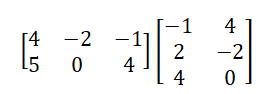#### Question 4 4. Which of the following is the slope of the given graph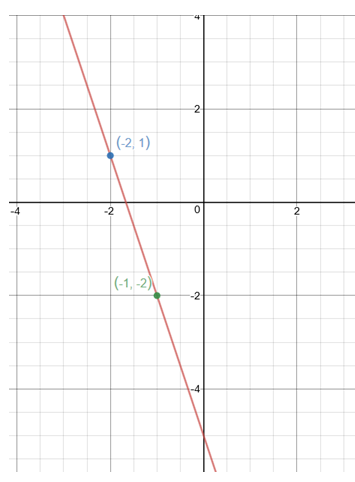#### Question 6 6. Two similar right circular cylinders have a scale factor of 3:4. What is the surface area of the largest right cylinder if the surface area of the smaller right cylinder is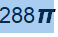#### Question 7 7. Which of the equations below is the correct expansions of the expression (r-3s)^3?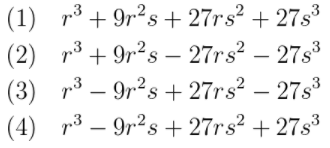#### Question 8 8. The numbers represented in the set below can all be classified as: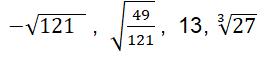#### Question 10 10. Evaluate